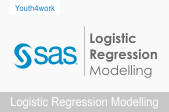SAS and Logistic Regression Modelling Course• ₹ 3999 75% オフ
₹ 999

This is an online course is to gain comprehensive understanding of SAS & Logistic Regression Modeling. The aim is to learn about how SAS & Logistic Regression Modeling and its features can be used. The tutorials will help you learn about the Regression Analysis, Predicting Probabilities, Logistics Regression and SAS Methodology.